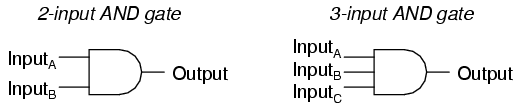### Lecture 4: Designing Circuits and operations and their gates (1)

26.06.12
DESIGNING CIRCUITS:

The first step in the design of a circuit is to establish a truth table that shows the output for all possible inputs.
Truth Tables
 not A A' and A B A.B or A B A+B 0 1 0 0 0 0 0 0 1 0 0 1 0 0 1 1 1 0 1 0 0 1 0 1 1 0 1 1 1 1 1 1

With proper input electronic digital circuits (logic circuits) establish logical manipulate paths. By passing binary signals through various combination of logic circuits, any desired information for computing can be operated on; each signal represents a binary carrying one "bit" of information.

The logic circuits or gates perform the logical operations.

OPERATIONS AND THEIR GATES:
1. AND GATEhttp://sub.allaboutcircuits.com/images/04100.pnghttp://sub.allaboutcircuits.com/images/04101.png
We can see, that Multiple input gates (gates with more than two inputs) exist in real world. Inverters and buffers exhaust the possibilities for single-input gate circuits. What more can be done with a single logic signal but to buffer it or invert it? To explore more logic gate possibilities, we must add more input terminals to the circuit(s). Adding more input terminals to a logic gate increases the number of input state possibilities. With a single-input gate such as the inverter or buffer, there can only be two possible input states: either the input is "high" (1) or it is "low" (0). As was mentioned previously in this chapter, a two input gate has four possibilities (00, 01, 10, and 11). A three-input gate has eight possibilities (000, 001, 010, 011, 100, 101, 110, and 111) for input states. The number of possible input states is equal to two to the power of the number of inputs:

Lets take example of multiple input AND gate:

Now above AND gate have three inputs and applying the formula of 2n, where n is number of inputs, with three inputs it becomes: 23=8, so we will have 8 possible rows in this truth table, in the pattern of (000 (FFF), 001(FFT), 010(FTF), 011(FTT), 100(TFF), 101(TFT), 110(TTF), and 111(TTT)). In this way we can construct truth tables for multiple input gates.
...continued

#### 1 comment:

1.QUANTUM BINARY SIGNALS

Get professional trading signals delivered to your cell phone every day.

Start following our signals right now and gain up to 270% a day.